# KSEEB SSLC Class 10 Maths Solutions Chapter 15 Surface Areas and Volumes Ex 15.4

KSEEB SSLC Class 10 Maths Solutions Chapter 15 Surface Areas and Volumes Ex 15.4 are part of KSEEB SSLC Class 10 Maths Solutions. Here we have given Karnataka SSLC Class 10 Maths Solutions Chapter 15 Surface Areas and Volumes Exercise 15.4.

## Karnataka SSLC Class 10 Maths Solutions Chapter 15 Surface Areas and Volumes Exercise 15.4

(Use π = $$\frac{22}{7}$$ unless stated otherwise.)

Question 1.
A drinking glass is in the shape of a frustum of a cone of height 14 cm. The diameters of its two circular ends are 4 cm and 2 cm. Find the capacity of the glass.
Solution:
Height of drinking glass, h = 14 cm.
Diameter of circular ends
D = 4 cm ∴ r = 2 cm.
d = 2 cm ∴ r = 1 cm.
Capacity of glass = ?Volume of glass = Radius with 2 cm + Volume of frustum with radius 1 cm.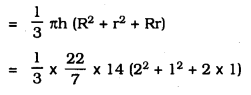Question 2.
The slant height of a frustum of a cone is 4 cm and the perimeters (circumference) of its ciruclar ends are 18 cm and 6 cm. Find the curved surface area of the frustum.
Solution:
Slant height of a i frustum of a cone, l = 4 cm.
Circumference of its circular ends = 18 cm. and 6 cm2πr =18 cm.
∴ R = $$\frac{9}{\pi}$$ cm.
2πr = 6
∴ r = $$\frac{3}{\pi}$$ cm.
∴ Lateral surface area of a frustum of a ConeQuestion 3.
A fez, the cap used by the Turks, is shaped like the frustum of a cone (see the figure).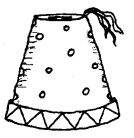If its radius on the open side is 10 cm, radius at the upper base is 4 cm, and its slant height is 15 cm, find the area of material used for making it.
Solution:
Radius on the open side of the cap, R = 10 cm.
Radius of upper end, r = 4 cm.
Slant height l = 15 cmR = 10 cm, r = 4 cm, l = 15 cm
∴ Curved surface = π × l × (R + r)
= π × 15 × (10 + 4)
= $$\frac{22}{7}$$ × 15 × 14
= 660 cm2
∴ Area of the cap at the end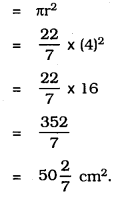∴ Area of material used for making it.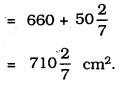Question 4.
A container, opened from the top and made up of a metal sheet, is in the form of a frustum of a cone of height 16 cm with radii of its lower and upper ends as 8 cm and 20 cm, respectively. Find the cost of the milk which can completely fill the container, at the rate of Rs. 20 per litre. Also find the cost of the metal sheet used to make the container, if it costs Rs. 8 per 100 cm2. (Take π = 3.14).
Solution:R = 20 cm
r = 8 cm
h = 20 cm
∴ Slant height,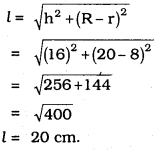∴ Volume of metallic sheet,Quantity of milk in the container
= $$\frac{10449.82}{1000}$$
Cost of 1 litre of milk is Rs. 20,
Cost of 10.45 litres of milk ……. ??
∴ 20 × 10.45 = Rs. 209.
Cost of metal sheet = π(R + r) + πr2
= π {20 × (20 + 8) + (8)2}
= 3.14 × 624
= 1959.36 cm2.
∴ Cost of preparing metallic container: For 100 cm2 Rs. 8
∴ For 1959.36 cm2 ……?
= 8 × $$\frac{1159.36}{100}$$
= Rs. 156.75.

Question 5.
A metallic right circular cone 20 cm high and whose vertical angle is 60° is cut into two parts at the middle of its height by a plane parallel to its base. If the frustum so obtained be drawn into a wire of diameter $$\frac{1}{16}$$ cm., find the length of the wire.
Solution:
Height of a cone, h = 20 cm.
Vertical angle = 60°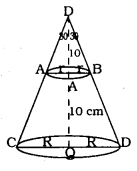Diameter of frustum wire = $$\frac{1}{16}$$ cm.
i) In ⊥∆AQC, ∠Q = 90°ii) In ⊥∆PRA, ∠R = 90°Height of frustum, h = 10 cm.
∴ Volume of metallic frustum, ABCD,Let the length of wire which has diameter $$\frac{1}{16}$$ cm be ‘x’ cm∴ Length of wire = 7964.4 m

We hope the given KSEEB SSLC Class 10 Maths Solutions Chapter 15 Surface Areas and Volumes Ex 15.4 will help you. If you have any query regarding Karnataka SSLC Class 10 Maths Solutions Chapter 15 Surface Areas and Volumes Exercise 15.4, drop a comment below and we will get back to you at the earliest.

error: Content is protected !!